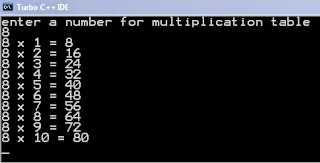Write a program in C++ for creating the multiplication table for the entered number. - WAY2ITCode :-

#include<iostream.h>
#include<conio.h>
//loops
//for loop
void main()
{
int a,n;
clrscr();
cout<<"enter a number for multiplication table"<<endl;
cin>>n;
for(a=1;a<=10;a++)
{
cout<<n<<" x "<<a<<" = "<<n*a<<endl;
}
getch();
}

Output :-